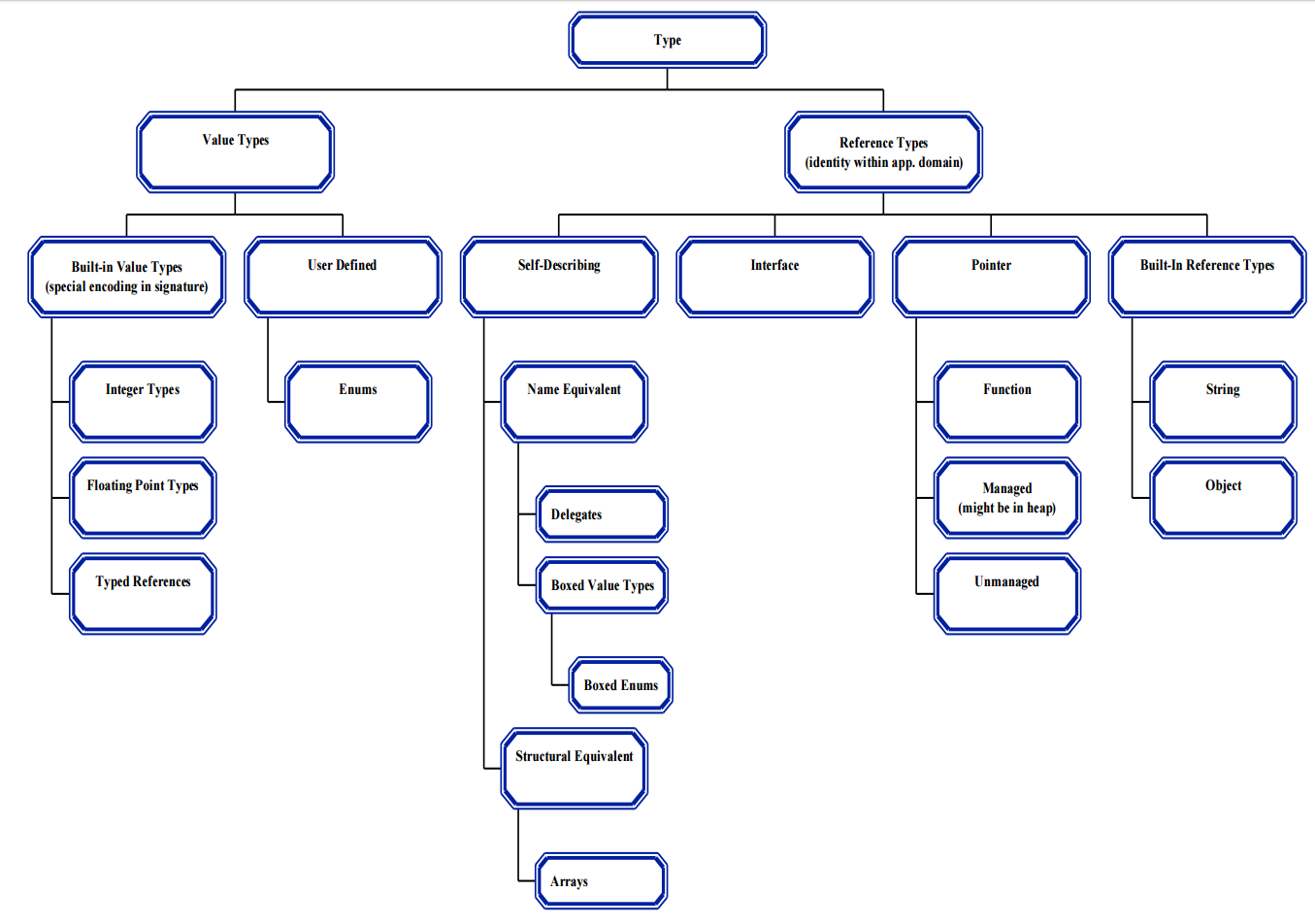# What is the difference between Reference Types and Value Types?

Here is where you will understand the difference between a Class and a Struct. Also the difference between Stack Memory and Heap Memory.

Before we talk about the differences between Reference Type and Value Type we need to understand some basic things.

## What is a data type?

A data type is a format which can contain some specific type or range of values. Basically, whenever you are declaring your variables you must assign some specific data type, as described here. On .NET Framework we have 2 data types: Reference Type and Value Type.

## Stack Memory and Heap Memory

There are 2 different types of memory we will talk about: Stack Memory and Heap Memory. While the Stack Memory receives the values using FILO (First In, Last Out) the Heap Memory is much more like an unorganized set of boxes in a room. This means that the access to the Stack Memory will be much faster than the Heap Memory. And here is the catch: The Stack Memory is where the Value Type stays. And the Heap Memory is where the Reference Type stays.

One thing to keep in mind: The Heap Memory is cleaned by the Garbage Collector, which means that Reference Types will be disposed automatically.

## What is Reference Type?

Reference Type is the type of data which makes a reference to where the value is placed.

A class is an example of reference type. If we copy a class into another class of the same type and change the values of the latter we will end up changing the values of the first one. This happens because the 2 classes are pointing to exactly the same place.

## What is Value Type?

Value Type is the type of data which holds the actual value of the object.

A struct is a good example of value type. If we follow the logic used above (copy a struct into another struct and change the values of the latter) we will realize that we didn’t change the value of the first struct. This happens because we literally copied the values from one object to another.

If you want to see it working make sure to go to my GitHub repository and download the code: https://github.com/davidsonsousa/Back-To-Basics/tree/master/ValueAndReferenceType